## Graphical analysis; and Vectors

9-13-99

Sections 2.8 - 3.4

### Graphs

Drawing good pictures can be the secret to solving physics problems. It's amazing how much information you can get from a diagram. We also usually need equations to find numerical solutions. Graphs are basically pictures of equations. Learning how to interpret these pictures can really help you understand physics.

Let's return to our last example, a ball thrown vertically upward with an initial speed of 12 m/s. The only acceleration we have to worry about is the acceleration due to gravity, 9.8 m/s2 down. This acceleration is constant, so it's easy to plot on a graph.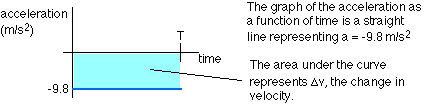If the time T represents the time when the ball returns to your hand, the area under the curve must equal -24.0 m/s, because we know the velocity changes from 12.0 m/s to -12.0 m/s. This allows us to solve for T, using:

T = -24.0 / -9.8 = 2.45 s, agreeing with what we calculated previously.

What about the velocity graph? The equation for velocity is:

v = vo + at
Plugging in the initial velocity and acceleration here gives v = 12.0 -9.8t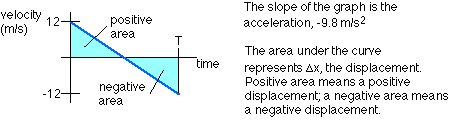The velocity graph can give all sorts of information:

• The slope of the velocity graph is the acceleration, while the area under the curve is the displacement.
• For this example of a ball going up and then back down, the graph confirms that the time taken on the way up equals the time taken on the way down.
• Calculating the area under the curve for the ball on the way up (the positive area on the graph) gives the maximum displacement. The area is just a triangle, with a a base T/2 = 1.2245 s and height of 12 m/s. This gives an area of 0.5(1.2245)(12) = 7.35 m. Again, this agrees with the maximum height calculated previously.
• The positive and negative areas cancel each other out, meaning the net displacement is zero. This is correct, as the ball returns to its starting point.

The graph of position as a function of time is a plot of the equation:

x = xo + vot + 1/2 at2
In this case, that's x = 0 + 12t -4.9t2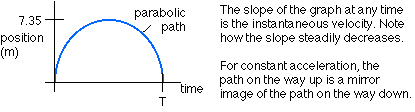The slope of the position graph gives the instantaneous velocity. This is positive but steadily decreasing on the way up, zero at the very top, and then becomes more and more negative on the way down.

### Vectors and scalars

We'll move on from looking at motion in one dimension to motion in two or three dimensions. It's critical now to distinguish between two kinds of quantities, scalars and vectors. A scalar is something that's just a number with a unit, like mass ( 200 g ) or temperature ( -30°C). A vector has both a number and a direction, like velocity. If you came to campus on the T today, at some point you may have been traveling 20 km/hr east. Velocity is a combination of a scalar (speed, 20 km/hr) and a direction (east).

Examples of scalars : mass, temperature, speed, distance

Examples of vectors : displacement, velocity, acceleration, force

One crucial difference between scalars and vectors involves the use of plus and minus signs. A scalar with a negative sign means something very different from a scalar with a plus sign; +30°C feels an awful lot different than -30°C, for example. With a vector the sign simply tells you the direction of the vector. If you're traveling with a velocity of 20 km/hr east, it means you're traveling east, and your speed is 20 km/hr. A velocity of -20 km/hr east also means that you're traveling at a speed of 20 km/hr, but in the direction opposite to east : 20 km/hr west, in other words. With a vector, the negative sign can always be incorporated into the direction.

Note that a vector will normally be written in bold, like this : A. A scalar, like the magnitude of the vector, will not be in bold face (e.g., A).

### Components of a vector

A vector pointing in a random direction in the x-y plane has x and y components: it can be split into two vectors, one entirely in the x-direction (the x-component) and one entirely in the y-direction (the y-component). Added together, the two components give the original vector.

The easiest way to add or subtract vectors, which is often required in physics, is to add or subtract components. Splitting a vector into its components involves nothing more complicated than the trigonometry associated with a right-angled triangle.

Consider the following example. A vector, which we will call A, has a length of 5.00 cm and points at an angle of 25.0° above the negative x-axis, as shown in the diagram. The x and y components of A, Ax and Ay are found by drawing right-angled triangles, as shown. Only one right-angled triangle is actually necessary; the two shown in the diagram are identical.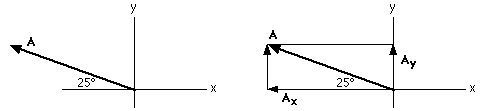Knowing the length of A, and the angle of 25.0°, Ax and Ay can be found by re-arranging the expressions for sin and cos.Note that this analysis, using trigonometry, produces just the magnitudes of the vectors Ax and Ay. The directions can be found by looking on the diagram. Usually, positive x is to the right of the origin; Ax points left, so it is negative:

Ax = -4.53 cm in the x-direction (or, 4.53 cm in the negative x-direction)

Positive y is generally up; Ay is directed up, so it is positive:

Ay = 2.11 cm in the y-direction

It's fairly easy to add vectors that are parallel to each other, or at right angles. In general, however, the angle between vectors being added (or subtracted) will be something other than 0, 90, or 180°. Consider the following example, where the vector C equals A + B.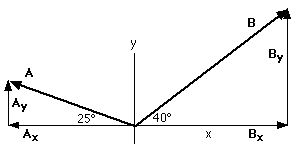A has a length of 5.00 cm and points at an angle of 25.0° above the negative x-axis. B has a length of 7.00 cm and points at an angle of 40.0° above the positive x-axis. If C = A + B, what is the magnitude and direction of C? There are basically two ways to answer this. One way is to draw a vector diagram, moving the tail of B to the head of A, or vice versa. The vector C will then extend from the origin to wherever the tip of the second vector is.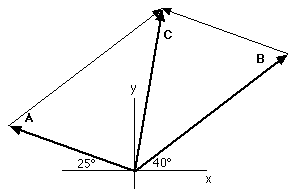The second way to find the magnitude and direction of C we'll use a lot in this course, because we'll often have vector equations of the form C =A + B. The simplest way to solve any vector equation is to split it up into one-dimensional equations, one equation for each dimension of the vector. In this case we're working in two dimensions, so the one vector equation can be replaced by two equations:

In the x-direction : Cx = Ax + Bx

In the y-direction : Cy = Ay + By

In other words, to find the magnitude and direction of C, the vectors A and B are split into components. The components are:

Ax = -4.532 cm in the x-direction

Ay = 2.113 cm in the y-direction

Bx = 7.00 cos40 = 5.362 cm

By = 7.00 sin40 = 4.500 cm

Bx = 5.362 cm in the x-direction

By = 4.500 cm in the y-direction

The components of C are found by adding the components of A and B:

Cx = Ax + Bx = (-4.532 + 5.362) cm in the x-direction = 0.83 cm in the x-direction

Cy = Ay + By= (2.113 + 4.500) cm in the y-direction = 6.61 cm in the y-direction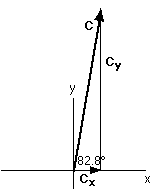The magnitude of C can be found from its components using the Pythagorean theorem: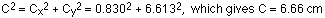The direction of C can be found by taking the inverse tangent of Cy/Cx:

inverse tan of 6.613 / 0.830 = 82.8°.

Combined, this gives C = 6.66 cm at an angle of 82.8° above the positive x-axis.

Note how the calculations and the diagrams go hand-in-hand. This will often be the case; it is always a good idea to draw diagrams as you go along.

Note also that we could have used the cosine law to get the length of C, and then applied the sine law, with a bit of geometry, to get the angle. It's worth trying that for yourself, just to convince yourself that the numbers come out the same.